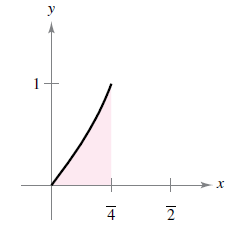Chapter 4.3, Problem 20E

Chapter
Section
Textbook Problem

Writing a Definite Integral In Exercises 13-22, write a definite integral that represents the area of the region. (Do not evaluate the integral.) f ( x ) = tan xTo determine

To calculate: Definite integral of f(x)=tanx representing the area of the provided region.

Explanation

Given: f(x)=tanx and, the region is:

Formula used:

On the closed interval [a,b], if f(x) is continuous and non-negative then the area of the region bounded by the graph of f(x), the x-axis, and the vertical lines x=a and x=b can be determined by:

Area=abf(x)dx

Calculation:

Using the given

Still sussing out bartleby?

Check out a sample textbook solution.

See a sample solution

The Solution to Your Study Problems

Bartleby provides explanations to thousands of textbook problems written by our experts, many with advanced degrees!

Get Started

Evaluate the integral. 13(x2+2x4)dx

Single Variable Calculus: Early Transcendentals, Volume I

In Exercises 2340, find the indicated limit. 38. limx22x3+4x2+1

Applied Calculus for the Managerial, Life, and Social Sciences: A Brief Approach

Sometimes, Always, or Never: limxax=0.

Study Guide for Stewart's Single Variable Calculus: Early Transcendentals, 8th

Identify the basic features of a between-subjects research design.

Research Methods for the Behavioral Sciences (MindTap Course List)

Identify possible sources and use them to identify a topic area for research.

Research Methods for the Behavioral Sciences (MindTap Course List)